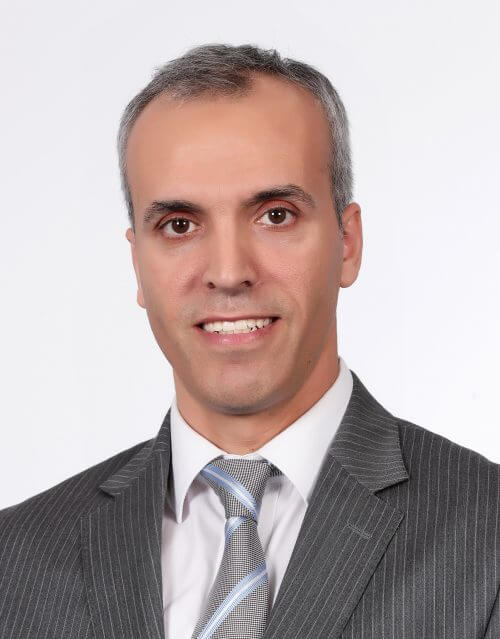Telephone +971 2 312 4086
Bio
Education
Teaching Areas
Research Interests
Bio

### Associate Professor, Department of Mathematics

Dr. Mohammad Al-Khaleel received an MSc in Numerical Analysis and a PhD in Scientific Computing-Numerical Analysis from McGill University, Montreal, QC, Canada, in 2003 and 2007, respectively.

In 2007, he became an assistant professor of mathematics with the Department of Mathematics, Yarmouk University, Jordan, and based on his achievements in research and teaching he was promoted to associate professor. In 2014, he took a one-year sabbatical leave to join Dhofar University in Oman as an associate professor of mathematics.

During his career, Dr. Al-Khaleel has published research papers in well-known journals such as SIAM Journal of Numerical Analysis and others. He also participated in many international conferences and spent several research periods at different places such as the Department of mathematics at University of Geneva, Switzerland.

He has been working on several projects on numerical solutions for differential equations using fast computations and efficient numerical algorithms including massive parallel algorithms for solving PDEs and large systems of ODEs such as those obtained from circuit simulations and from semi-discretized PDEs. In addition, he has been also working on projects on inverse and control problems, electronic circuit mathematical models, numerical parameter optimization, and projects on the existence of fixed points and coincidence points for contractive mappings in metric spaces; the usual metric space and its variants spaces such as partial, G-metric, and G-cone metric.

Education
• PhD in Applied Mathematics, McGill University, Canada
• MSc in Applied Mathematics McGill University, Canada
• BSc in Mathematics, Yarmouk University, Jordan
Teaching Areas
• Calculus I
• Calculus II
• Calculus III
• Engineering Mathematics
• Differential Equations and Linear Algebra
• Complex variables with applications
• Numerical Analysis I
• Numerical Analysis II
• Mathematics for Pre-Med Program
Research Interests
• Efficient and parallel numerical algorithms for solving differential equations
• Circuit simulations and computer arithmetic
• Functional analysis and fixed point theory
• Numerical solutions of differential equations
• Inverse and control problems
• Electronic circuit mathematical models
• Numerical parameter optimization
• Contractive mappings as well as fixed points